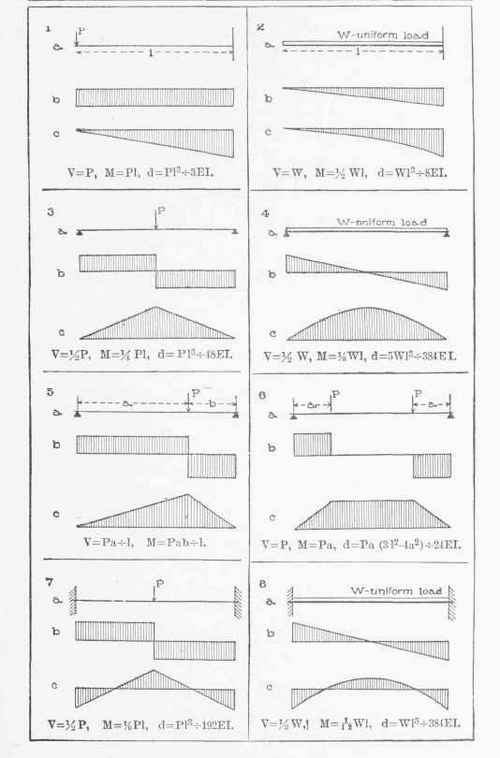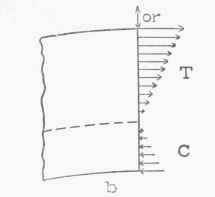56. Kinds of Loads Considered. The loads that are applied to a horizontal beam are usually vertical, but sometimes forces are applied otherwise than at right angles to beams. Forces acting on beams at right angles are called transverse forces; those applied parallel to a beam are called longitudinal forces; and others are called inclined forces. For the present we deal only with beams subjected to transverse forces (loads and reactions).

## Table B

Shear Diagrams (b) and Moment Diagrams (c) for Eight Different Cases (a). Also Values of Maximum Shear (V), Bending Moment (M), and Deflection (d).57. Neutral Surface, Neutral Line, and Neutral Axis. When a beam is loaded it may be wholly convex up (concave down), as a cantilever; wholly convex down (concave up), as a simple beam on end supports; or partly convex up and partly convex down, as a simple beam with overhanging ends, a restrained beam, or a continuous beam. Two vertical parallel lines drawn close together on the side of a beam before it is loaded will not be parallel after it is loaded and bent. If they are on a convex-down portion of a beam, they will be closer at the top and farther apart below than when drawn (Fig. 32a), and if they are on a convex-up portion, they will be closer below and farther apart above than when drawn (Fig. 325).Fig. 32.

The "fibres" on the convex side of a beam are stretched and therefore under tension, while those on the concave side are shortened and therefore under compression. Obviously there must be some intermediate fibres which are neither stretched nor shortened, i.e., under neither tension nor compression. These make up a sheet of fibres and define a surface in the beam, which surface is called the neutral surface of the beam. The intersection of the neutral surface with either side of the beam is called the neutral line, and its intersection with any cross-section of the beam is called the neutral axis of that section. Thus, if ah is a fibre that has been neither lengthened nor shortened with the bending of the beam, then nn is a portion of the neutral line of the beam; and, if Fig. 32c be taken to represent a cross-section of the beam, NN is the neutralaxis of the section.

It can be proved that the neutral axis of any cross-section of a loaded beam passes through the center of gravity of that section, provided that all the forces applied to the beam are transverse, and that the tensile and compressive stresses at the cross-section are all within the elastic limit of the material of the beam.

58. Kinds of Stress at a Cross=section of a Beam. It has already been explained in the preceding article that there are tensile and compressive stresses in a beam, and that the tensions are on the convex side of the beam and the compressions on the concave (see Fig. 33). The forces T and C are exerted upon the portion of the beam represented by the adjoining portion to the right (not shown). These, the student is reminded, are often called fibre stresses.Fig. 33.

Besides the fibre stresses there is, in general, a shearing stress at every cross-section of a beam. This may be proved as follows:

Fig. 34 represents a simple beam on end supports which has actually been cut into two parts as shown. The two parts can maintain loads when in a horizontal position, if forces are applied at the cut ends equivalent to the forces that would act there if the beam were not cut. Evidently in the solid beam there are at the section a compression above and a tension below, and such forces can be applied in the cut beam by means of a short block C and a chain or cord T, as shown. The block furnishes the compressive forces and the chain the tensile forces. At first sight it appears as if the beam would stand up under its load after the block and chain have been put into place. Except in certain cases*, however, it would not remain in a horizontal position, as would the solid beam. This shows that the forces exerted by the block and chain (horizontal compression and tension ) are not equivalent to the actual stresses in the solid beam. What is needed is a vertical force at each cut end.

* When the external shear for the section is zero.

Suppose that R1 is less than L1 + L2 + weight of A, i. e., that the external shear for the section is negative; then, if vertical pulls be applied at the cut ends, upward on A and downward on B, the beam will stand under its load and in a horizontal position, just as a solid beam. These pulls can be supplied, in the model of the beam, by means of a cord S tied to two brackets fastened on A andFig. 34.Fig. 35.

B, as shown. In the solid beam the two parts act upon each other directly, and the vertical forces are shearing stresses, since they act in the plane of the surfaces to which they are applied.

59. Relation Between the Stress at a Section and the Loads and Reactions on Either Side of It. Let Fig. 35 represent the portion of a beam on the left of a section; and let R1 denote the left reaction; L1 and L2 the loads; W the weight of the left part;

C, T, and S the compression, tension, and shear respectively which the right part exerts upon the left.

Since the part of the beam here represented is at rest, all the forces exerted upon it are balanced; and when a number of horizontal and vertical forces are balanced, then

1. The algebraic sum of the horizontal forces equals zero.